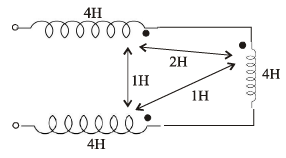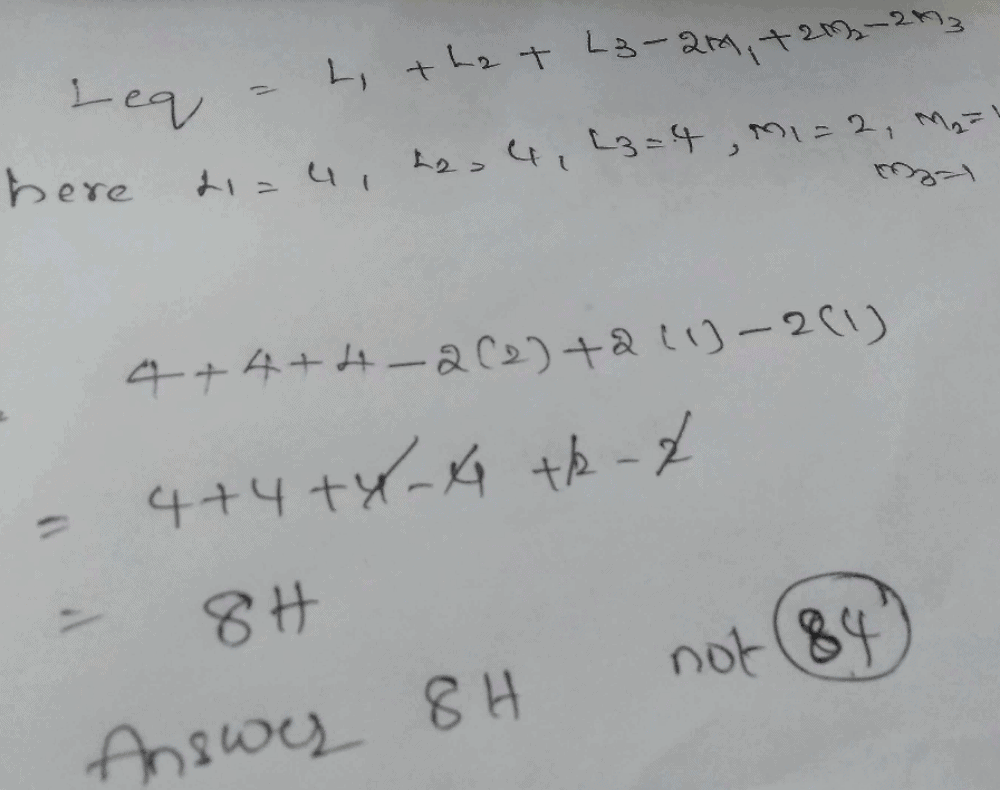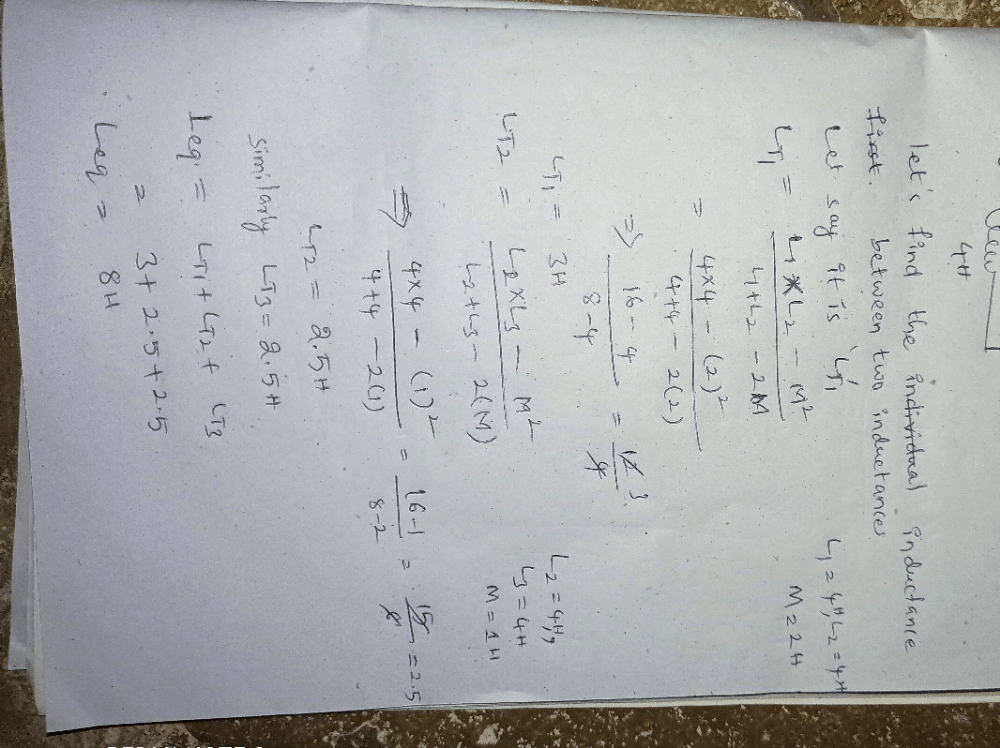Courses

# The equivalent inductance at seen at terminal AB is_____a)164b)104c)84d)124Correct answer is option 'C'. Can you explain this answer? Related Test: Technical Test SSC JE: Electrical Engineering (EE)- 1

## Electrical Engineering (EE) QuestionPyata Nagraj Jun 25, 2019Shiv Mangal Apr 11, 2020
C ,84Aryan Kumar Apr 19, 2020Asim Farheen Jul 02, 2020
C: 84.Raja Rathnam Apr 08, 2020
84Kommukuri V Rani Sep 16, 2020
If it is connected in series combination let we have to imagine it can be divided as 3
so
Lt=L1+L2+L3
I.,e LT= 3*28=84Saravanan Saravanan Nov 24, 2020
104Gm Ram Nov 25, 2020
Pagl h kya iska ans 8 aayega kon h qn dene valaShiv . Nov 03, 2020
M1, M2, M3 are the mutual inductances.
Leq= L1+L2+L3
�2M1
�2M2
�2M3
The sign conventions depend upon the dots. If dots of both mutual inductors are at positve/negative potential
like dot of L1 is at +ve terminal while the dot of L2 is at +ve terminal
then addition is done & if the dots of both mutual inductors are at either potential, like dot of L1 is at +ve terminal while the dot of L2 is at -ve terminal then subtraction is done.Lingam Pranitha Sep 30, 2020
DTakshshila Choudhary Sep 22, 2020
C 85Suraj Kumar Nov 16, 2020
Option c is correct because we can using induction in series and parallel combinationThirupathi Mandha Feb 09, 2020
Ans 8HRikitha Volle Oct 11, 2020Sourabh Patil Jun 03, 2020
8

This discussion on The equivalent inductance at seen at terminal AB is_____a)164b)104c)84d)124Correct answer is option 'C'. Can you explain this answer? is done on EduRev Study Group by Electrical Engineering (EE) Students. The Questions and Answers of The equivalent inductance at seen at terminal AB is_____a)164b)104c)84d)124Correct answer is option 'C'. Can you explain this answer? are solved by group of students and teacher of Electrical Engineering (EE), which is also the largest student community of Electrical Engineering (EE). If the answer is not available please wait for a while and a community member will probably answer this soon. You can study other questions, MCQs, videos and tests for Electrical Engineering (EE) on EduRev and even discuss your questions like The equivalent inductance at seen at terminal AB is_____a)164b)104c)84d)124Correct answer is option 'C'. Can you explain this answer? over here on EduRev! Apart from being the largest Electrical Engineering (EE) community, EduRev has the largest solved Question bank for Electrical Engineering (EE).

### Related Content

This discussion on The equivalent inductance at seen at terminal AB is_____a)164b)104c)84d)124Correct answer is option 'C'. Can you explain this answer? is done on EduRev Study Group by Electrical Engineering (EE) Students. The Questions and Answers of The equivalent inductance at seen at terminal AB is_____a)164b)104c)84d)124Correct answer is option 'C'. Can you explain this answer? are solved by group of students and teacher of Electrical Engineering (EE), which is also the largest student community of Electrical Engineering (EE). If the answer is not available please wait for a while and a community member will probably answer this soon. You can study other questions, MCQs, videos and tests for Electrical Engineering (EE) on EduRev and even discuss your questions like The equivalent inductance at seen at terminal AB is_____a)164b)104c)84d)124Correct answer is option 'C'. Can you explain this answer? over here on EduRev! Apart from being the largest Electrical Engineering (EE) community, EduRev has the largest solved Question bank for Electrical Engineering (EE).